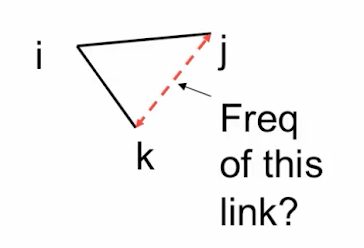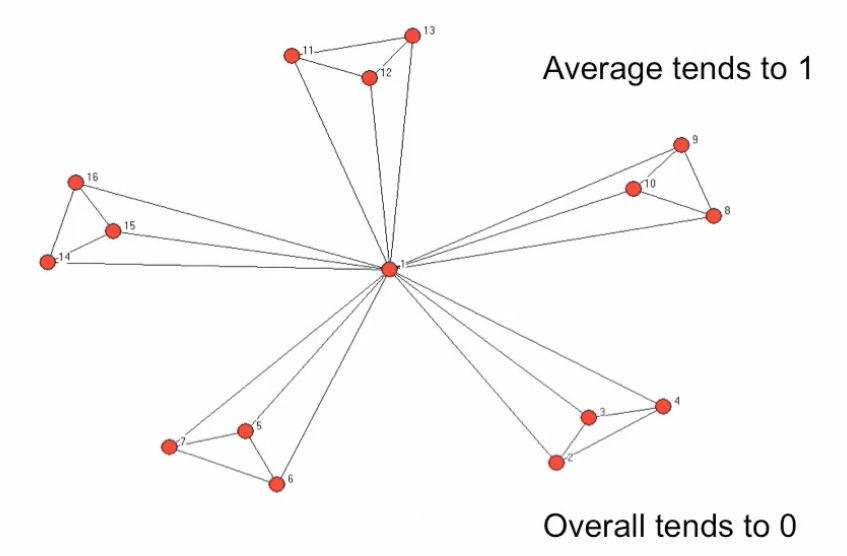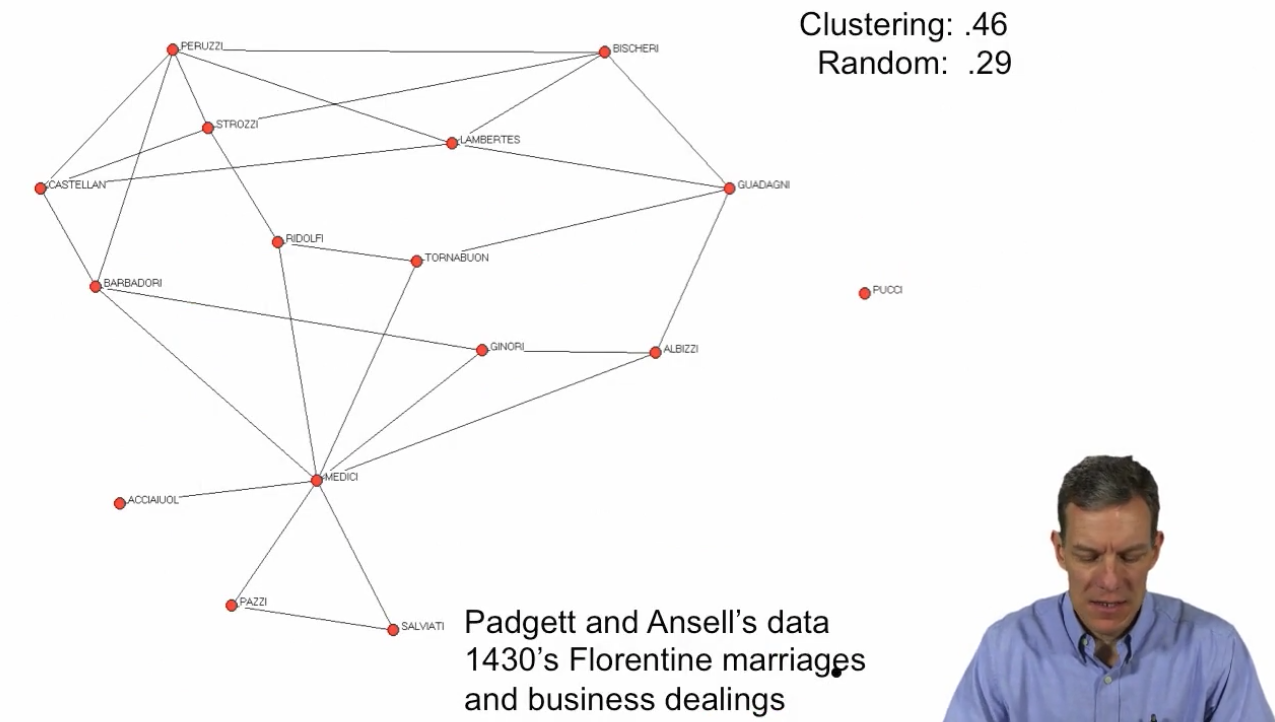# Clustering¶• What fraction of my friends are friends of each other?

• $Cl_i(g)$ = #{kj in g | k, j in $N_i(g)$} / #{kj | k, j in $N_i(g)$}

• Average clustering: $$Cl^{avg}(g) = \sum_i Cl_i(g)/n$$

• Overall clusterig:

• $Cl(g)$ = $\sum_i$ #{kj in g | k, j in $N_i(g)$} / $\sum_i$ #{kj | k, j in $N_i(g)$}# Clustering in a Poisson Random Network¶

• Average and Overall clustering tend to 0, if max degree is bounded and network becomes larege:

• $Cl(g)$ = $\sum_i$ #{kj in g | k, j in $N_i(g)$} / $\sum_i$ #{kj | k, j in $N_i(g)$} is simply p
• If degree is bounded, then p(n-1) is bounded

• So p goes to 0 as n grows

# High? Clustering coefficients¶

• Prison friendships

• 0.31 (MacRae 60) vs 0.0134 (G(n, p) model)
• CO-authorships

• 0.15 math (Grossman 02) vs 0.00002
• 0.09 biology (Newman 01) vs 0.00001
• 0.19 econ(Goyal et al 06) vs 0.00002
• www

• 0.11 for web links (Adamic 99) vs 0.0002